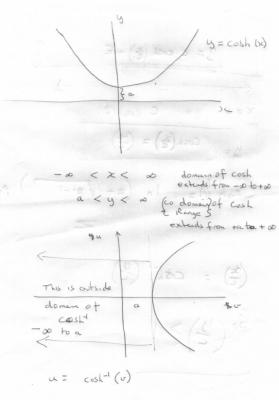# Catenary solution problem solving for constant 'a' in cosh formula.

## Recommended Posts

I am a new member & have a problem, the subject of which has interested me for years. The solution for a in the hyperbolic function (for a catenary)

y = aCosh(x/a), sometimes with +c added as a 'curve shifter'.

I have managed in the past by iterative trial and error if y & x are known, but very time consuming, ineligant, sometimes unsuccessful. The Casio calculator I use has a "solver", which is useful when it can find an answer, but often can't. I have a new all singing & dancing HP 50G with several solvers, which is far to complicated for me to use at all!

The problem is me. I do not understand why sometimes a cannot be found? Surely every Cosh curve must have an a, in fact the thing always has y=1 when x=0, the a being a 'scaling factor' to define what part of the curve is to be considered for span, sag, height above ground etc for a symmetrical portion of it. When a is negative the curve inverts, having vanished while passing through y=0.

Is there a way of transposing for a - (I can't do it in the simple formula above)?

What sort of equation is it anyway, not linear, not polynomial, not entirely logarithmic? So what 'solver' should be looking at it?

Please do not ask me to rush into Calculus. Tried it in my youth, can't do it. Sorry for my inepitude. I'm sure this is the right place however to admit this, and to ask for any help, for which I would be most interested & grateful.

##### Share on other sites

It is not clear how you are solving your equations.

However there are restrictions on the domain of the inverse hyperbolic cosine in particular for any w

${\cosh ^{ - 1}}\left( w \right) = \ln \left( {w + \sqrt {{w^2} - 1} } \right):w \ge 1$

Given these restrictions let us consider your equation

$y = a\cosh \left( {\frac{x}{a}} \right)$

$\frac{x}{a} = {\cosh ^{ - 1}}\left( {\frac{y}{a}} \right)$

$\left( {\frac{y}{a}} \right) \ge 1$

$y \ge a$

Edited by studiot

##### Share on other sites

Thank you Studiot for your reply. There is much food for thought for me here, especially in the choosing of the values to be in the equation. Quite obviously, given your answer, some combinations just can't work.

I am much obliged to you for this info, I can even understand it! Regards Mike.

##### Share on other sites

What are you using your catenaries for?

Do you have a derivation for my logarithmic formula and have you seen the alternative secant solution to the catenary?

##### Share on other sites

Thanks for asking! Not using them for anything, but am just fascinated by the beauty of the shape in power lines, washing lines, etc. An obsessive and totally esoteric interest, perhaps the fallout of an engineering degree 65 years ago. Soothing to the mind, a continued defiance to dementure perhaps. (Except when the blighters wont solve).

And no, Studiot I do not have your derivation of your logarithmic formula, and probably I would not understand a word; I will however gladly take your word for the most informative result you have given above. The 'alternative secant solution' does sound very tempting however...

But I must'nt keep you, Regards Mike_B.

##### Share on other sites

Let $x = {\cosh ^{ - 1}}\left( w \right)$

Then $w = \cosh \left( x \right)$

So $\sinh \left( x \right) = \sqrt {{w^2} - 1}$

Therefore ${e^x} = w + \sqrt {{w^2} - 1}$

Take logs

$x = \ln \left( {w + \sqrt {{w^2} - 1} } \right) = {\cosh ^{ - 1}}\left( w \right)$

as required.

The attachment shows the domain of cosh(x) and its inverseThe arc length, is geven as a function of the slope, theta and a.

$s = a\tan \theta$

differentiate

$\frac{{ds}}{{d\theta }} = a{\sec ^2}\theta$

also

$\frac{{dx}}{{ds}} = \cos \theta$

So $\frac{{dx}}{{d\theta }} = \frac{{dx}}{{ds}} \bullet \frac{{ds}}{{d\theta }}$

$\frac{{dx}}{{d\theta }} = \cos \theta a{\sec ^2}\theta = a\sec \theta$

Integrate

$x = a\int {\frac{{d\theta }}{{\cos \theta }}} = a\ln \left( {\sec \theta + \tan \theta } \right)$

Similarly

$\frac{{dy}}{{d\theta }} = \sin \theta a{\sec ^2}\theta$

Integrate

$y = a\int {\frac{{\sin \theta d\theta }}{{{{\cos }^2}\theta }}} = a\sec \theta + D$

Which gives x and y as functions of the slope.

Constants c and D can be made zero by suitable choice of coordinate axes, as with the other solution.

##### Share on other sites

Most interesting. Will give me some new slants on solving, and on the general theory of solution limitations. And the diagram of both curves together will make things much clearer. Thoughtful of you.

And I still have asymmetric catenaries to come!

I am very touched by your unstinting & prompt help, and to Science Forums for extending such a wealth of knowledge to those in need.

Regards Mike_B.

##### Share on other sites

I noticed a small error in line 3 of post#4.

Perhaps some kind mod would put it right.

$\sinh (w) = \sqrt {{w^2} - 1}$

Of course it should be noted that these equations only apply when the cable is uniformly loaded throughout its length.

This is sometimes the case but not for engineering structures such as bridges and cable stayed/supported structures.

Telephone wires and some washing lines are heavy enough for their own weight to be sufficient loading, but for lines that approach weightless the curve approaches more and more nearly a parabola.

The above is the mathematical approach, a good treatment of the engineering approach to simplify things is given in

Pippard

"Analysis of Engineering Structures"

Edited by studiot

##### Share on other sites

Thanks for this lead re Pippard. Have updated my printout with the small correction mentioned above.

Nice to have corresponded with you, Mike_B.

## Create an account

Register a new account